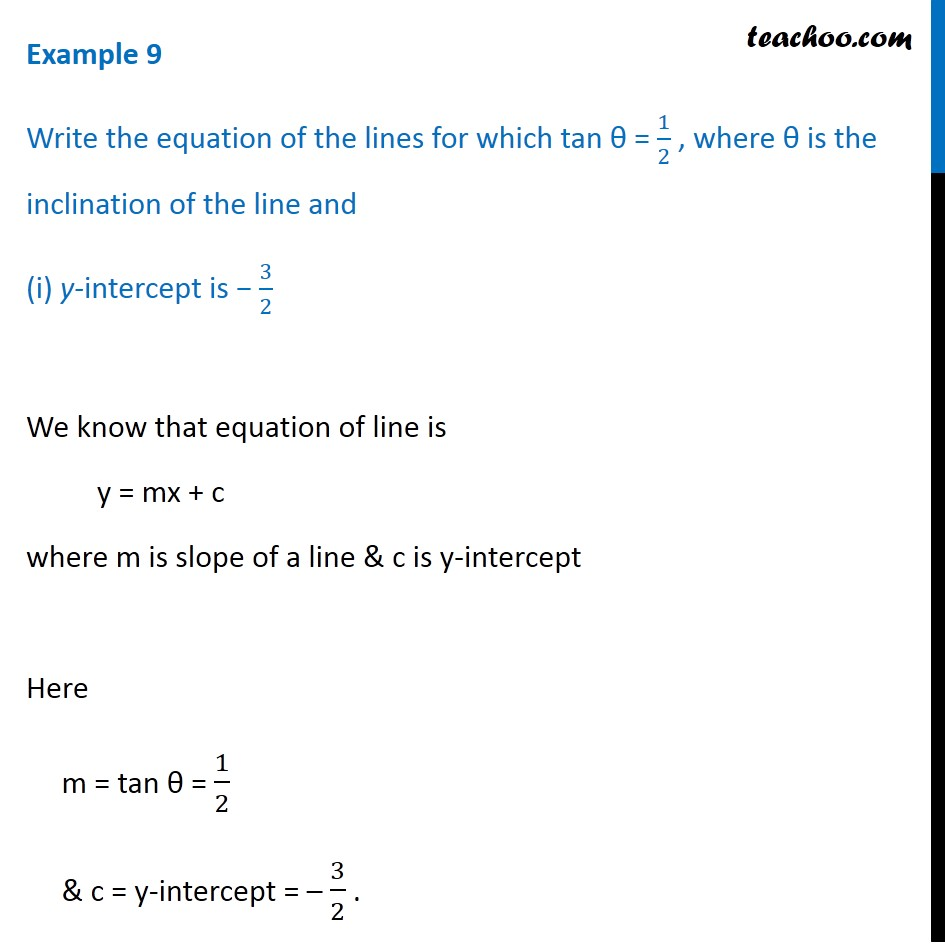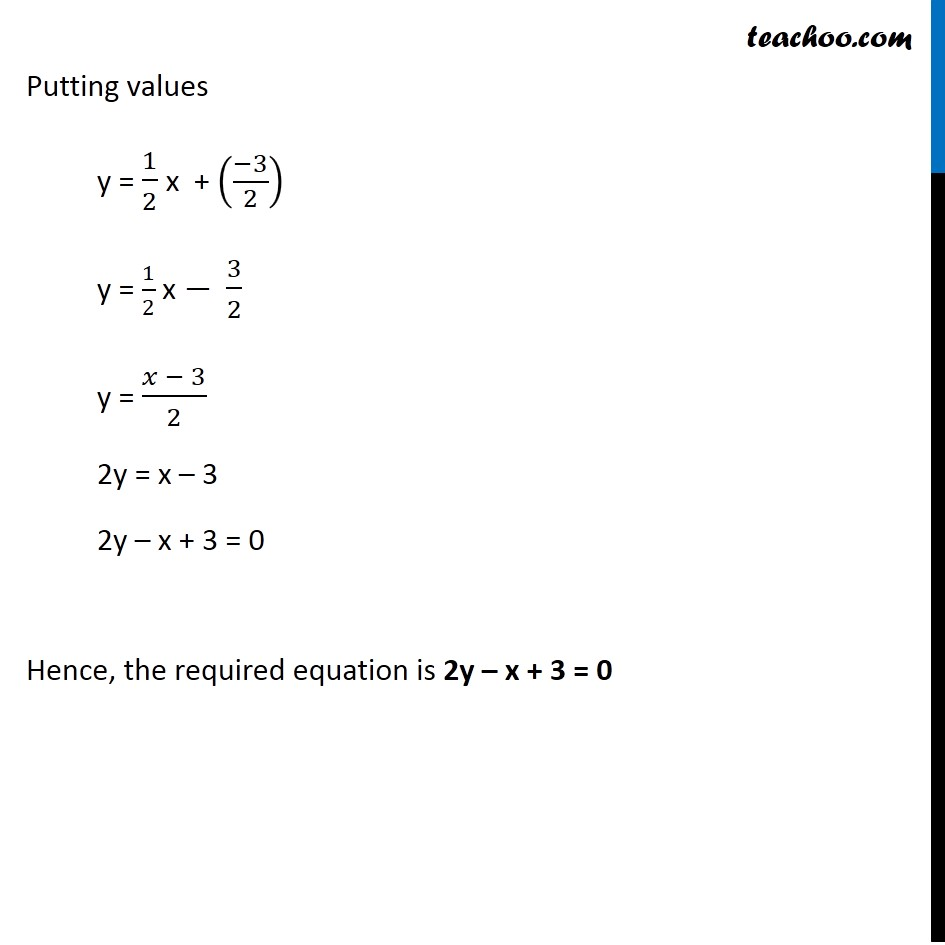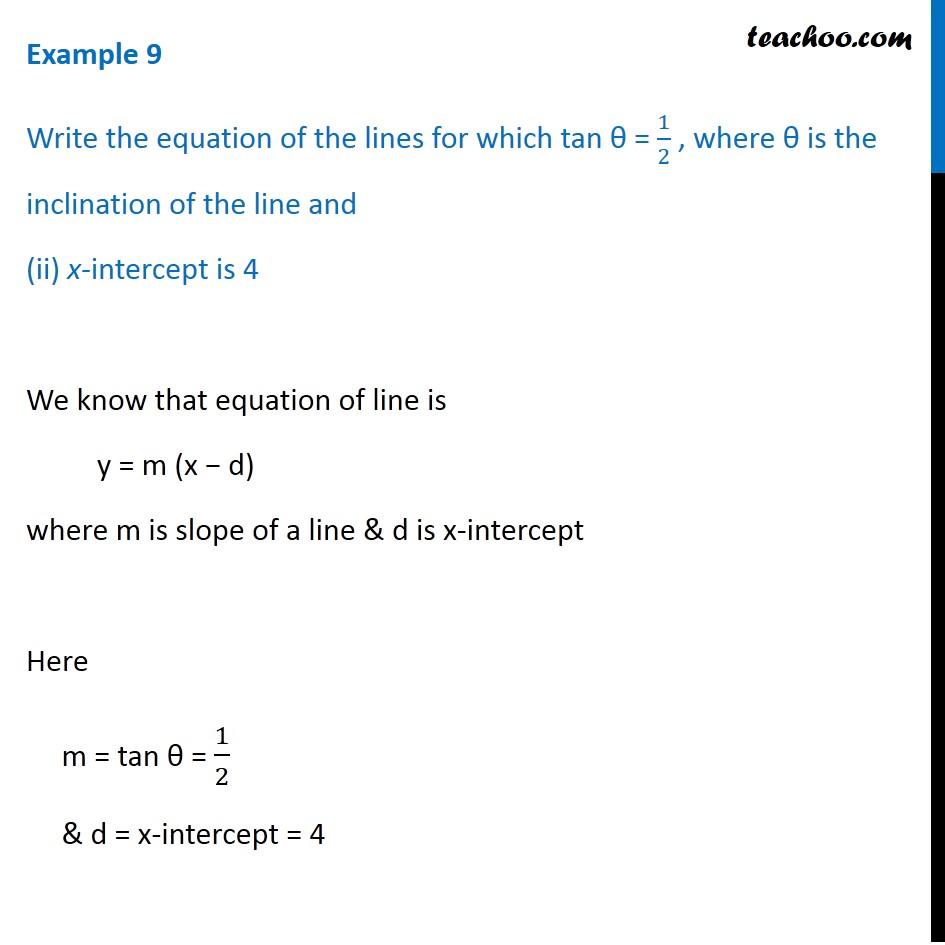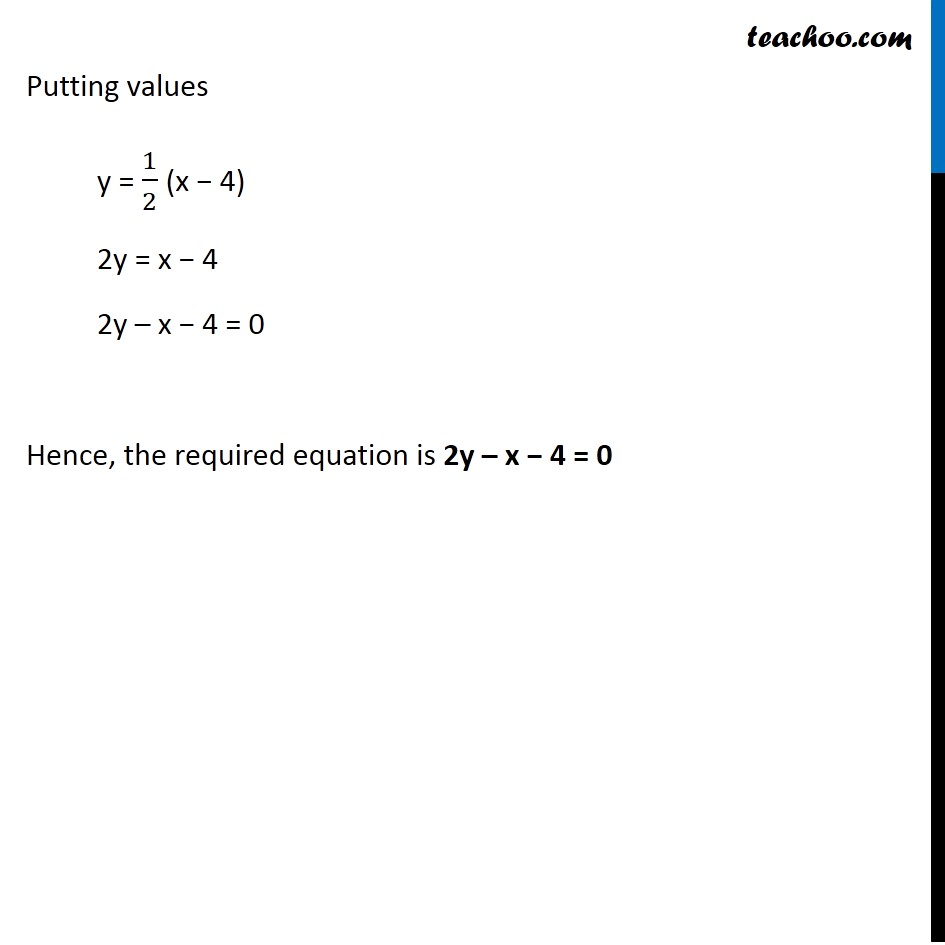Subscribe to our Youtube Channel - https://you.tube/teachoo

1. Chapter 10 Class 11 Straight Lines
2. Serial order wise
3. Examples

Transcript

Example 9 Write the equation of the lines for which tan θ = 1/2 , where θ is the inclination of the line and (i) y-intercept is − 3/2 We know that equation of line is y = mx + c where m is slope of a line & c is y-intercept Here m = tan θ = 1/2 & c = y-intercept = – 3/2 . Putting values y = 1/2 x + ((−3)/2) y = 1/2 x − 3/2 y = (𝑥 − 3)/2 2y = x – 3 2y – x + 3 = 0 Hence, the required equation is 2y – x + 3 = 0 Example 9 Write the equation of the lines for which tan θ = 1/2 , where θ is the inclination of the line and (ii) x-intercept is 4 We know that equation of line is y = m (x − d) where m is slope of a line & d is x-intercept Here m = tan θ = 1/2 & d = x-intercept = 4 Putting values y = 1/2 (x − 4) 2y = x − 4 2y – x − 4 = 0 Hence, the required equation is 2y – x − 4 = 0

Examples